## Example Questions

2 Next →

### Example Question #221 : Arithmetic

Find the decimal equivalent of the percentage: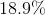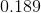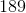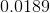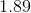Explanation:

In order to find the decimal equivalent of a percentage, the number that makes up the percent has to be divided by 100. However, since it is division by a power of 10, we can accomplish the same thing, by moving the decimal point 2 places to the left, thus making the number smaller. For this problem, that looks like this: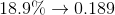### Example Question #222 : Arithmetic

Find the decimal equivalent to the percentage: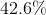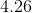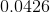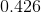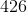Explanation:

In order to find the decimal equivalent of a percentage, the number that makes up the percent has to be divided by 100. However, since it is division by a power of 10, we can accomplish the same thing, by moving the decimal point 2 places to the left, thus making the number smaller. For this problem, that looks like this: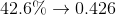### Example Question #51 : Percentage

Find the decimal equivalent of the percentage: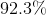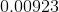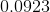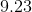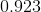Explanation:

In order to find the decimal equivalent of a percentage, the number that makes up the percent has to be divided by 100. However, since it is division by a power of 10, we can accomplish the same thing, by moving the decimal point 2 places to the left, thus making the number smaller. For this problem, that looks like this: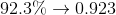### Example Question #224 : Arithmetic

Find the decimal equivalent of the percentage: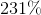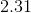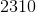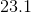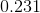Explanation:

In order to find the decimal equivalent of a percentage, the number that makes up the percent has to be divided by 100. However, since it is division by a power of 10, we can accomplish the same thing, by moving the decimal point 2 places to the left, thus making the number smaller. For this problem, that looks like this: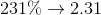### Example Question #225 : Arithmetic

Find the decimal equivalent of the percentage: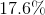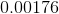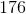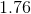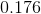Explanation:

In order to find the decimal equivalent of a percentage, the number that makes up the percent has to be divided by 100. However, since it is division by a power of 10, we can accomplish the same thing, by moving the decimal point 2 places to the left, thus making the number smaller. For this problem, that looks like this:### Example Question #226 : Arithmetic

Find the decimal equivalent of the percentage: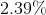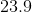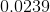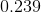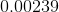Explanation:

In order to find the decimal equivalent of a percentage, the number that makes up the percent has to be divided by 100. However, since it is division by a power of 10, we can accomplish the same thing, by moving the decimal point 2 places to the left, thus making the number smaller. For this problem, that looks like this: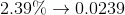2 Next →

### All SAT Math Resources